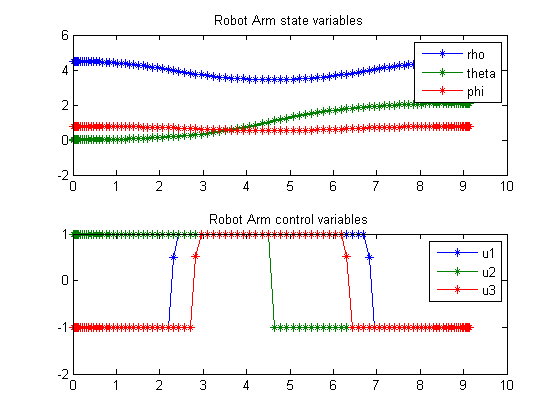93  Robot Arm Movement

Benchmarking Optimization Software with COPS Elizabeth D. Dolan and Jorge J. More ARGONNE NATIONAL LABORATORY

93.1  Problem Formulation

Find u(t) over t in [0; 1 ] to minimize

 J = tf

subject to:

L*
 d2rho dt2
= u1
I1*
 d2theta dt2
= u2
I2*
 d2phi dt2
= u3

 0 <= rho <= L
 |theta| <= pi
 0 <= phi <= pi
 |u1:3| <= 1

I1 =
 ((L−rho)3+rho3) 3
*sin(phi)2
I2 =
 ((L−rho)3+rho3) 3

The boundary conditions are:

[rho0  theta0  phi0] = [4.5  0
 pi 4
[rho1  theta1  phi1] = [4.5
 2*pi 3

 pi 4
 L = 5

All first order derivatives are 0 at boundaries.

Reference: 

93.2  Problem setup

toms t
toms t_f

% Initial guess
tfopt = 1;
x1opt = 4.5;
x2opt = 0;
x3opt = 2*pi/3*t.^2;
x4opt = 0;
x5opt = pi/4;
x6opt = 0;
u1opt = 0;
u2opt = 0;
u3opt = 0;

for n=[20 100]
%rho d(rho)dt theta d(theta)dt phi d(phi)dt
p = tomPhase('p', t, 0, t_f, n);
setPhase(p);
tomStates x1 x2 x3 x4 x5 x6
tomControls u1 u2 u3

% Initial guess
x0 = {t_f == tfopt
icollocate({x1 == x1opt
x2 == x2opt; x3 == x3opt
x4 == x4opt; x5 == x5opt
x6 == x6opt})
collocate({u1 == u1opt
u2 == u2opt; u3 == u3opt})};

% Box constraints
L = 5;
cbox = {
0.1 <= t_f <= 10
0   <= icollocate(x1) <= L
-pi <= icollocate(x3) <= pi
0   <= icollocate(x5) <= pi
-1  <= collocate(u1)  <= 1
-1  <= collocate(u2)  <= 1
-1  <= collocate(u3)  <= 1};

% Boundary constraints
cbnd = {initial({x1 == 4.5; x2 == 0
x3 == 0; x4 == 0
x5 == pi/4; x6 == 0})
final({x1 == 4.5; x2 == 0
x3 == 2*pi/3
x4 == 0
x5 == pi/4
x6 == 0
})};

I1 = ((L-x1).^3+x1.^3)./3.*sin(x5).^2;
I2 = ((L-x1).^3+x1.^3)/3;

% ODEs and path constraints
ceq = collocate({dot(x1) == x2
dot(x2) == u1/L;   dot(x3) == x4
dot(x4) == u2./I1; dot(x5) == x6
dot(x6) == u3./I2});

% Objective
objective = t_f;

93.3  Solve the problem

options = struct;
options.name = 'Robot Arm';
solution = ezsolve(objective, {cbox, cbnd, ceq}, x0, options);

% Optimal x, y, and speed, to use as starting guess in the next iteration
x1opt = subs(x1, solution);
x2opt = subs(x2, solution);
x3opt = subs(x3, solution);
x4opt = subs(x4, solution);
x5opt = subs(x5, solution);
u1opt = subs(u1, solution);
u2opt = subs(u2, solution);
u3opt = subs(u3, solution);
tfopt = subs(final(t), solution);
Problem type appears to be: lpcon
Starting numeric solver
===== * * * =================================================================== * * *
TOMLAB - Tomlab Optimization Inc. Development license  999001. Valid to 2011-02-05
=====================================================================================
Problem: ---  1: Robot Arm                      f_k       9.146367545948111300
sum(|constr|)      0.000000273543612574
f(x_k) + sum(|constr|)      9.146367819491723900
f(x_0)      1.000000000000000000

Solver: snopt.  EXIT=0.  INFORM=1.
SNOPT 7.2-5 NLP code
Optimality conditions satisfied

FuncEv    1 ConstrEv   17 ConJacEv   17 Iter   11 MinorIter  225
CPU time: 0.125000 sec. Elapsed time: 0.125000 sec.
Problem type appears to be: lpcon
Starting numeric solver
===== * * * =================================================================== * * *
TOMLAB - Tomlab Optimization Inc. Development license  999001. Valid to 2011-02-05
=====================================================================================
Problem: ---  1: Robot Arm                      f_k       9.140854009735486200
sum(|constr|)      0.000002639183837389
f(x_k) + sum(|constr|)      9.140856648919323000
f(x_0)      9.146367545948111300

Solver: snopt.  EXIT=0.  INFORM=1.
SNOPT 7.2-5 NLP code
Optimality conditions satisfied

FuncEv    1 ConstrEv    7 ConJacEv    7 Iter    4 MinorIter  729
CPU time: 1.812500 sec. Elapsed time: 1.875000 sec.
end

t  = subs(collocate(t),solution);
x1 = subs(collocate(x1),solution);
x3 = subs(collocate(x3),solution);
x5 = subs(collocate(x5),solution);
u1 = subs(collocate(u1),solution);
u2 = subs(collocate(u2),solution);
u3 = subs(collocate(u3),solution);

93.4  Plot result

subplot(2,1,1)
plot(t,x1,'*-',t,x3,'*-',t,x5,'*-');
legend('rho','theta','phi');
title('Robot Arm state variables');

subplot(2,1,2)
plot(t,u1,'*-',t,u2,'*-',t,u3,'*-');
legend('u1','u2','u3');
title('Robot Arm control variables');